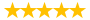# keller proj586 Week 3 Homework AIB Problems

Question # 00003565 Posted By: neil2103 Updated on: 11/15/2013 07:56 AM Due on: 11/27/2013
Subject General Questions Tutorials:
Question

Problem 1

The following data were obtained from a project to create a new portable electronic.

Activity Duration Predecessors

A 5 Days ---

B 6 Days ---

C 8 Days ---

D 4 Days A, B

E 3 Days C

F 5 Days D

G 5 Days E, F

H 9 Days D

I 12 Days G

Step 1: Construct a network diagram for the project.

Step 2: Answer the following questions: (15 points total)

a) What is the Scheduled Completion of the Project? (5 points)

b) What is the Critical Path of the Project? (5 points)

c) What is the ES for Activity D? (1 points)

d) What is the LS for Activity G? (1 points)

e) What is the EF for Activity B? (1 points)

f) What is the LF for Activity H? (1 points)

g) What is the float for Activity I? (1 points)

Problem 2

The following data were obtained from a project to build a pressure vessel:

Activity Duration Predecessors

A 6 weeks ---

B 6 weeks ---

C 5 weeks B

D 4 weeks A, C

E 5 weeks B

F 7 weeks D, E, G

G 4 weeks B

H 8 weeks F

I 5 weeks G

J 3 week I

Step 1: Construct a network diagram for the project.

Step 2: Answer the following questions: (15 points total)

a) Calculate the scheduled completion time. (5 points)

b) Identify the critical path (6 points)

c) What is the slack time (float) for activity A? (1 points)

d) What is the slack time (float) for activity D? (1 points)

e) What is the slack time (float) for activity E? (1 points)

f) What is the slack time (float) for activity G? (1 points)

Problem 3

The following data were obtained from a project to design a new software package:

Activity Duration Predecessors

A 5 Days ---

B 8 Days ---

C 6 Days A

D 4 Days C, B

E 5 Days A

F 4 Days D, E, G

G 4 Days B, C

H 3 Day G

Step 1: Construct a network diagram for the project.

Step 2: Answer the following questions: (15 points total)

a) Calculate the scheduled completion time. (5 points)

b) Identify the critical path(s) (6 points)

c) What is the slack time (float) for activity B? (1 points)

d) What is the slack time (float) for activity D? (1 points)

e) What is the slack time (float) for activity E? (1 points)

f) What is the slack time (float) for activity G? (1 points)

Problem 4

The following data were obtained from an in-house MIS project:

Activity Duration Predecessors

A 5 Days ---

B 8 Days ---

C 5 Days A

D 4 Days B

E 5 Days B

F 3 Day C, D

G 7 Days C, D

H 6 Days E, F, G

I 9 Days E, F

Step 1: Construct a network diagram for the project.

Step 2: Answer the following questions: (15 points total)

a) Calculate the scheduled completion time. (5 points)

b) Identify the critical path (6 points)

c) What is the slack time (float) for activity A? (1 points)

d) What is the slack time (float) for activity D? (1 points)

e) What is the slack time (float) for activity E? (1 points)

f) What is the slack time (float) for activity F? (1 points)

Tutorials for this Question
1. ## Solution: keller proj586 Week 3 Homework AIB Problems

Tutorial # 00003370 Posted By: neil2103 Posted on: 11/15/2013 07:57 AM
Puchased By: 8
Tutorial Preview
The solution of keller proj586 Week 3 Homework AIB Problems...
Attachments
Week_3_Homework_AIB_Problems.docx (23 KB)
Recent Feedback
Rated By Feedback Comments Rated On
jo...ettPlagiarism-free work 06/22/2016
ca...pc1Convenient and satisfactory work 06/24/2015
ta...olyDelivery before the deadline 03/02/2015
mo...samExpert tutors 02/25/2015
ik...ingProvide assignment and homework help in all subjects 12/18/2013

Great! We have found the solution of this question!